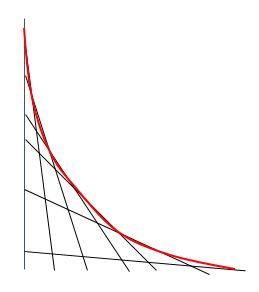# Tangential Curve

Calculus Level 5Let $t \in [0, 1]$ be a parameter. Let $\ell_t$ be the line passing through the points $(t,0)$ and $(0,1-t)$.

For each value of $a$ we can define the point $P_t$ where $\ell_t$ intersects the neighboring lines $\ell_{t+dt}$.

The collection of points $P_t$ traces out a curve, as suggested in the drawing above. This curve satisfies the equation $x^n + y^n = 1$. Determine the value of $n$.

×